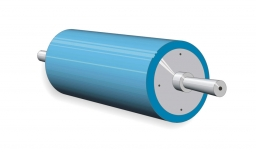# Perimeter of base

The circumference of the base of the rotating cylinder is same as its height. What is the diameter and height of this cylinder with volume 1 liter?

Correct result:

D =  7.4004 cm
h =  23.2489 cm

#### Solution:We would be pleased if you find an error in the word problem, spelling mistakes, or inaccuracies and send it to us. Thank you!Tips to related online calculators
Do you know the volume and unit volume, and want to convert volume units?

#### You need to know the following knowledge to solve this word math problem:

We encourage you to watch this tutorial video on this math problem:

## Next similar math problems:

• CircleWhat is the radius of the circle whose perimeter is 6 cm?
• MineWheel in traction tower has a diameter 4 m. How many meters will perform an elevator cabin if wheel rotates in the same direction 89 times?
• Circular poolThe 3.6-meter pool has a depth of 90 cm. How many liters of water is in the pool?
• FlowerbedIn the park there is a large circular flowerbed with a diameter of 12 m. Jakub circulated him ten times and the smaller Vojtoseven times. How many meters each went by and how many meters did Jakub run more than Vojta?
• A pipeA radius of a cylindrical pipe is 2 ft. If the pipe is 17 ft long, what is its volume?
• Common cylinderI've quite common example of a rotary cylinder. Known: S1 = 1 m2, r = 0.1 m Calculate : v =? V =? You can verify the results?
• Simplify 2Simplify expression: 5ab-7+3ba-9
• Find theFind the radius of the circular base of the vessel, whose perimeter is 2.51 m. Write the result to one decimal place in meters. Via the π sign - Ludolph's number
• Circle r,DCalculate the diameter and radius of the circle if it has length 26.59 cm.
• ConvaHow many liters of water fit into the shape of a cylinder with a bottom diameter 20 cm and a height 45 cm?
• The potDiameter of the pot 38 cm. The height is 30 cm. How many liters of water can fit in the pot?
• Collect rain waterThe garden water tank has a cylindrical shape with a diameter of 80 cm and a height of 12 dm. How many liters of water will fit into the tank?
• Cylindrical tankCylindrical tank holds 600hl water and is deep 2.5 m. Calculate the diameter of the cylinder.
• BottleA company wants to produce a bottle whose capacity is 1.25 liters. Find the dimensions of a cylinder that will be required to produce this 1.25litres if the hight of the cylinder must be 5 times the radius.
• Tank and waterCylindrical tank were poured with 3.5 liters of water. If tank base diameter is 3 dm, how height is water level in?
• ContainerThe container has a cylindrical shape the base diameter 0.8 m and the area of the base is equal to the area of the wall. How many liters of water can we pour into the container?
• The potThe pot is a cylinder with a volume of V = 7l and an inner diameter of d = 20cm. Find its depth.